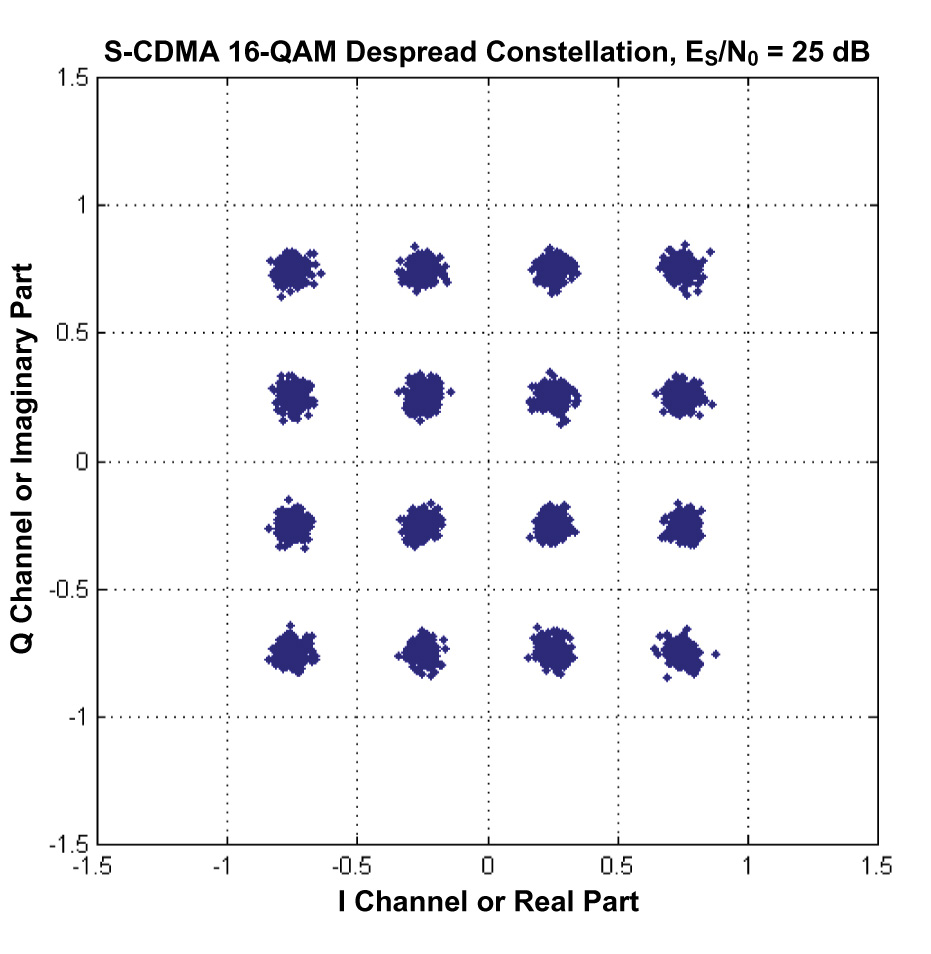﻿ QAM MODULATION?Back

## QAM MODULATION?QAM stands for Quadrature Amplitude Modulation. It is digital modulation technique. This modulation technique is a combination of both Amplitude and phase modulation techniques. QAM is better than QPSK in terms of data carrying capacity. QAM takes benefit from the concept that two signal frequencies one shifted by 90 degree with respect to the other can be transmitted on the same carrier. For QAM, each carrier is ASK modulated hence at different phases, further the data can be carried at different amplitudes .

As mentioned for each symbol both phase and amplitudes are varied to represent different bits. There are two levels of amplitudes for each phase d1 and d2. There are many variants to this technique. Most popular are 16-QAM, 64-QAM and 256-QAM. The example below explains 16-QAM. In 16-QAM each symbol represents 4 bits as mentioned in the constellation diagram below.
For example if the input is 1010 then the output is (-3-j*3)*KMOD.
Typically KMOD is 1/root (10) for 16-QAM.In 64-QAM, each symbol is represented by 6 bits and in 256-QAM, each symbol is represented by 8 bits. As it increases in level QAM technique becomes more bandwidth efficient but it requires very robust algorithms to decode complex symbol at receiver into bits. For example 256-QAM is complex than 16-QAM. QAM is more bandwidth efficient compare to BPSK but it is less robust. Hence for better CINR in the system QAM is employed which leads better data rate. For poor CINR BPSK is employed. CINR stands for Carrier to Interference and Noise Ratio.